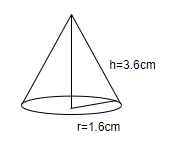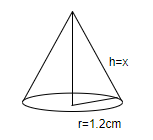QUESTION

# A right circular cone is 3.6cm high and the radius of its base is 1.6cm. It is melted and recast into a right circular cone with radius of its base as 1.2cm. Find its height. (a) 6.4cm(b) 5.4cm(c) 6.8cm(d) 4.4cm

Hint: Calculate the volume of the right circular cone using the formula $\dfrac{1}{3}\pi {{r}^{2}}h$, where ‘r’ is the radius of the cone and ‘h’ is the height of the cone. Substitute $r=1.6cm,h=3.6cm$ in the above formula to calculate the volume of the cone. Assume that the height of the new cone is ‘x’. Substitute $r=1.2cm,h=x$ in the above formula to calculate the volume of the new cone. Equate the volume of both the cones and simplify the equation by rearranging the terms to calculate the height of the new cone.

We know that a right circular cone with radius equal to 1.6cm and height equal to 3.6cm is melted into a cone of radius 1.2cm. We have to calculate the height of this cone.
We know that the volume of the right circular cone is $\dfrac{1}{3}\pi {{r}^{2}}h$, where ‘r’ is the radius of the cone and ‘h’ is the height of the cone.
Substituting $r=1.6cm,h=3.6cm$ in the above formula, the volume of the cone is $=\dfrac{1}{3}\pi {{\left( 1.6 \right)}^{2}}\left( 3.6 \right)$.Simplifying the above expression, the volume of the cone $=\dfrac{1}{3}\pi {{\left( 1.6 \right)}^{2}}\left( 3.6 \right)=9.64608c{{m}^{3}}$.
We will now calculate the volume of the cone whose radius is 1.2cm. Let’s assume that the height of this cone is ‘x’.
Substituting $r=1.2cm,h=x$ in the above formula, the volume of the new cone $=\dfrac{1}{3}\pi {{\left( 1.2 \right)}^{2}}x$.Simplifying the above expression, the volume of the new cone $=\dfrac{1}{3}\pi {{\left( 1.2 \right)}^{2}}x=1.5072xc{{m}^{3}}$.

We know that the volume of both the cones is equal. Thus, we have $9.64608=1.5072x$.
Rearranging the terms of the above equation, we have $x=\dfrac{9.64608}{1.5072}=6.4cm$.
Hence, the height of the new cone is 6.4cm, which is option (a).

Note: When one cone is melted to form a new cone, the volume of both the cones must be equal. We should be careful about units while calculating the volume of the cone. As the dimensions of the cone are given in centimetres, the volume of the cone will be in cubic centimetres and thus, the height of the cone will be in centimetres again.